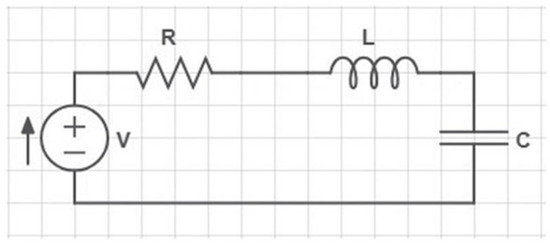Next Article in Journal
The 3-Rainbow Domination Number of the Cartesian Product of Cycles
Previous Article in Journal
Conformal Equitorsion and Concircular Transformations in a Generalized Riemannian Space
Previous Article in Special Issue
Mathematical Analysis of a Thermostatted Equation with a Discrete Real Activity Variable
Open AccessArticle

# Fixed-Point Results for a Generalized Almost (s, q)—Jaggi F-Contraction-Type on b—Metric-Like Spaces

byHasanen A. Hammad 1andManuel De la Sen 2,*1
Department of Mathematics, Faculty of Science, Sohag University, 82524 Sohag, Egypt
2
Institute of Research and Development of Processes, University of the Basque Country, 48940 Leioa (Bizkaia), Spain
*
Author to whom correspondence should be addressed.
Mathematics 2020, 8(1), 63; https://doi.org/10.3390/math8010063
Received: 15 October 2019 / Revised: 14 December 2019 / Accepted: 17 December 2019 / Published: 2 January 2020
The purpose of this article is to present a new generalized almost $( s , q ) −$ Jaggi $F −$ contraction-type and a generalized almost $( s , q ) −$ Jaggi $F −$ Suzuki contraction-type and some results in related fixed point on it in the context of $b −$ metric-like spaces are discussed. Also, we support our theoretical results with non-trivial examples. Finally, applications to find a solution for the electric circuit equation and second-order differential equations are presented and an strong example is given here to support the first application. View Full-Text
Show FiguresFigure 1

MDPI and ACS Style

Hammad, H.A.; De la Sen, M. Fixed-Point Results for a Generalized Almost (s, q)—Jaggi F-Contraction-Type on b—Metric-Like Spaces. Mathematics 2020, 8, 63.